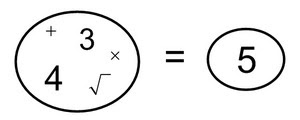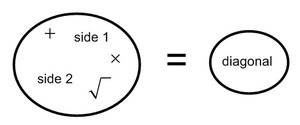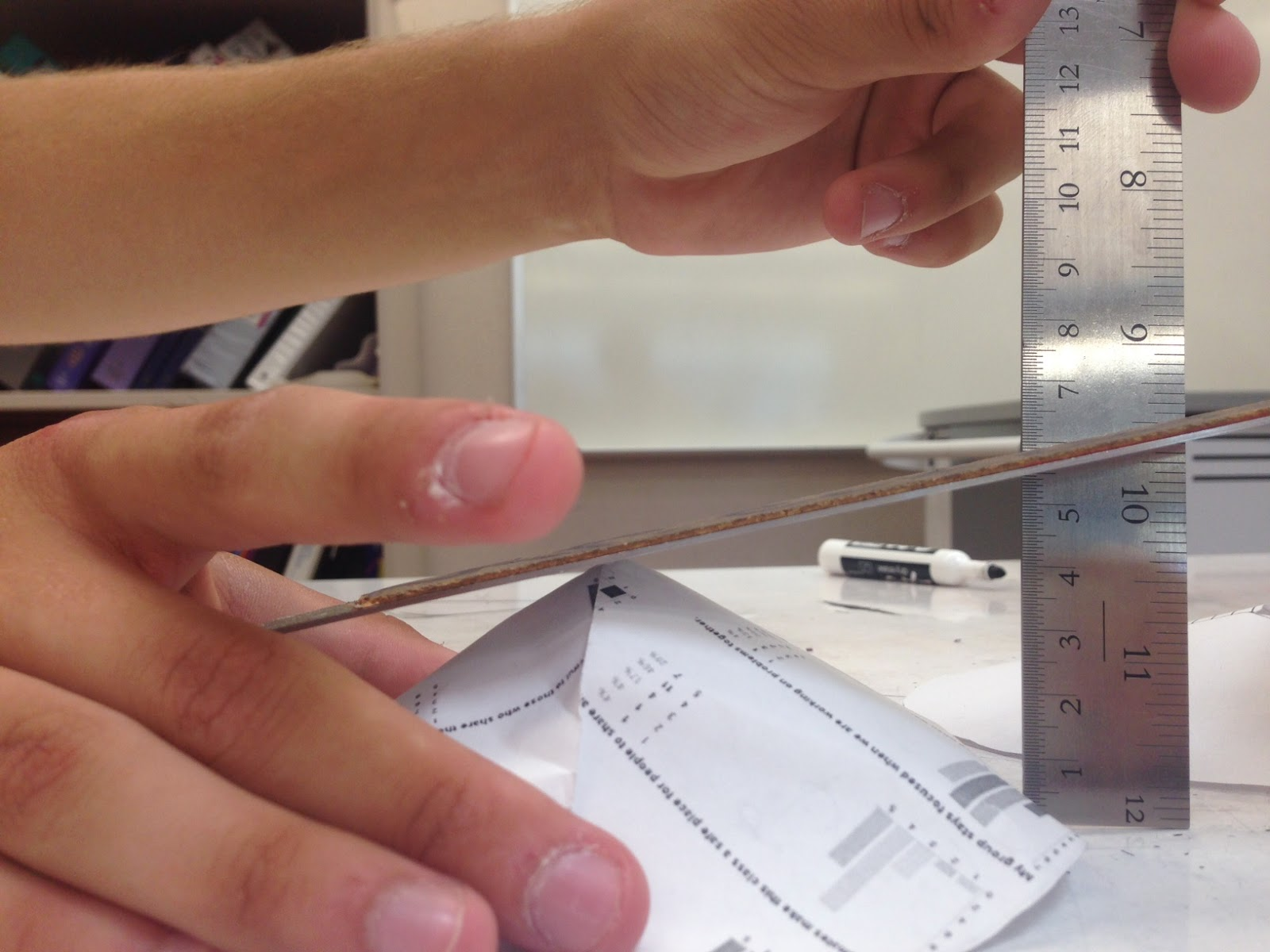## Tuesday, August 12, 2014

### Question: How can we find the diagonal length of any rectangle?

Part 1:  On a sheet of paper, draw the following rectangles:
A.  3 x 4
B.  5 x 12
C.  6 x 8
D. 8 x 15
Part 2:  Draw the diagonal for each rectangle.
Part 3:  Measure the length of each diagonal and record it in the table below.
 Rectangle Side 1 Side 2 Diagonal A 3 4 5 B 5 12 C 6 8 D 8 15

Part 4: Use the side lengths of the rectangle, addition, multiplication and square root to write an expression that equals the diagonal length.
Rectangle 1 would look like this:Be sure that your equation works for each of the rectangles.
Part 5:  Write a general equation that could be used for any rectangle.Part 6: Draw a new a x b rectangle.  Use your equation from part 5 to predict what the diagonal length should be.
Part 7: How accurate was your prediction?  Explain.

### Commentary

This task asks students to notice a special relationship between the sides of rectangle and its diagonal, which they can later apply to the sides of a right triangle and its hypotenuse.  It is the numeric counterpart to task Sizing Up Squares, which looks at the same relationship geometrically.  Students will draw rectangles of given side lengths, measure the diagonals, and then find an equation that relate the sides to the diagonal using specified operations: addition, multiplication, and square roots. Once students have an equation that works for the initial data, they will write a general equation, and test this hypothesis against other rectangles of their choosing.
This task allows students to construct right triangles indirectly (in the context of rectangles and diagonals), observe the relationship between their side lengths, and then "play" with the numbers and use inductive reasoning to discover the Pythagorean relationship.
Teacher Note:  (1) Units are not specified here so there is flexibility in how students construct the rectangles.  If graph paper is used, students may need guidance with regard to measuring the diagonal lengths.  For example: if using the unit of the graph paper to construct the sides of the rectangles but using a ruler to measure the diagonals, they will need to divide the ruler measurements by the size of the grid to ensure that the numbers being compared are in the same unit.  Alternatively, they could use a strip of the same graph paper as the "ruler," with the unit of measurement being the side length of each square on the paper. (2) Use of calculators may facilitate students' experimentation with the numbers in Part 4.

## Friday, May 9, 2014

### Well, since you asked...

We've been looking at the volume of prisms, cylinders and cones this week.  I had students working on a project where they had to build one of each with equal heights and widths/diameters.  The idea is to explore the volume of each and see how the eventual formulas will relate to one another.

Then, Jacob traces can on his paper and cuts out the circle.  He cuts a radius and begins rolling the paper (as if he's cut out different sized sectors) to make different cones.  He comes up and says, "Mr. Cox,  I think the cone that is almost flat has the highest volume because the tighter I roll the paper, the less stuff I can fit in it."

Me:  What if the circle is flat?  What's the volume then?

J: There isn't any volume.

Me:  So then when does the cone go from 'flat' to having the most possible volume?

J:  ...

Me: ...

J: What do you mean?

Me:  Maybe there's some kind of sweet spot where the volume gets bigger then starts to get smaller.

J:  Let me think about that.

At this point, I was with Jacob.  I didn't really know what the volume did as the cone changed.  But we were both interested.

The next day, Jacob comes in and says, "Mr. Cox, I thought about what you were saying and I think you're right, there has to be some kind of sweet spot."

So, we sit down and go to work.

I'm thinking about how to model this thing and Jacob enlisted the help of a friend to gather data.  They're cutting sectors from a circle and making cones.  Jacob has dibs on 30, 60, 90, ... degrees and Armando has 15, 45, 75, ...

Our first bit of trouble came when Jacob said, "I can find the radius of the cone's base, but I'm having trouble getting the height because of this..."Wish these rulers came with a bubble level.

But, we figured out that the Pythagorean Theorem was a nice work around.

Now, does our data match the model?

It took a while, and thanks to CalcDave for cleaning things up, but this is a pretty cool function.

Desmos graph is here.

We're estimating the maximum to be about 66 degrees.  And because my calculus is a little rusty, I'm thankful for the folks at WolframAlpha.This particular function is using a circle with radius = 3.1.

The function is a little dense at this point, but Jacob was dialed in as we talked about it.  The idea that these crazy expressions really just amounted to Vcone = ⅓πr2h blew him away.

## Tuesday, May 6, 2014

### Full Circle

It was one of those moments when I was trying to explain something to them and they ended up explaining something to me.

We're in the middle of a unit on volume and exploring prisms, cylinders and cones.  I was inspired by James Tanton's ability to explain things by getting at their essence. As if to say, "we can call a cylinder a 'cylinder' but it's just a prism made of circles--or a cone can be called a 'cone' but is it really any different than a pyramid?"

It was one of those, sitting around a campfire moments.  We're using stacks of paper and stacks of CDs to demonstrate why calculating the base area is critical because the rest of the solid is just like a stack of that area and no matter where we slice the solid, we get the same shape--over and over again.

Then comes the question about the cone.

The base is a circle but when you slice it, you get a...circle?  Wait, but it's a different circle.  Waitaminit. What about a pyramid?  Triangle base and when you slice it, you get a triangle.  But a different one.

Are the triangles related?

"They're similar.  Hey wait, this is a dilation."

And the tip of the pyramid is the center of dilation.

We did dilations in Unit 1.  This was a callback I didn't anticipate:  A pyramid is like a 3D representation of a dilation.

Thanks, kids.  I'd never thought of it that way before.

### I'm Taking You With Me

I've applied for one of our district coaching positions.  There are still a lot of details to work out, so I'm not quite sure I'm ready to leave the classroom.  One thing I am sure of, though, is that I'd like the interview panel (assuming I get an interview) to understand  how amazing you all are.

I tweeted this form, but I'll leave it here as well.  If you have a minute, I'd love your help.

Feel free to exclude any information you're not interested in sharing.

Thanks a bunch.

## Friday, May 2, 2014

### When It Can't Be Wrecked

We're getting some mileage out of this lately.  Today, I have a new problem to add to the pile of those that foster the process of hypothesis wrecking.

I posed the question with a rubric.

Can a unit fraction always be written as the sum of two unique unit fractions?

Rubric
5: Precise proof that demonstrates all cases (abstract, general rule)
4: Reasonable argument that demonstrates some cases (numeric, gives examples)
3: Gut level or weak argument
2: Does not present an argument

1: No evidence of understanding

Students played around with a few unit fractions and after a few minutes we had a couple of them.

Shortly, we had a student come up with an hypothesis:

which was soon followed by another student example:

Uh-oh, that doesn't fit the pattern.

"Does this example wreck our hypothesis?"

This led to a nice conversation on whether this new example and our hypothesis can coexist.  It was interesting to see how many students initially thought the hypothesis was wrecked.

We tested a few more examples and shared results--all confirming our hypothesis.

Then I asked, "So where does this put us on the rubric?"

And a student asks, "What has to happen for a 4 to become a 5?"

In other words, when does a numeric (quantitative) argument become abstract ?

Had to pause.  This one is worth it.  So we discussed simple example:

I quickly came up with the question and answers 1, 3, and 4.  At lunch I added 2, which really added to the conversation for 6th period.

Which answer provides the stronger argument?  Most saw 4 as the strongest and agreed 1 was the weakest.  But very few saw 2 on the same level as 4.  Then one student says, "I see that 2 and 4 are similar but 4 is just kinda strung out."

Yep, the kid has a feel for brute force vs. elegance.  Love it.

By the end, we agreed that 2 and 4 were more abstract and 3 was more quantitative. What about 1?

Well, 1 was what they would've considered a great answer a few months ago.

 This is what prompted my question about SMP 2 on Twitter.

## Wednesday, April 16, 2014

### Dirty Triangles

I've been out for a couple of days--let's just say that I can think of better ways to drop 10 pounds--so, I'm in a really special frame of mind today.  While I was out, I left a few distance/rate/time problems for students to solve.  Upon my return, I was asking students about the problems and many students had similar responses.

S:  "This is easy, you just use the Dirt Triangle."

Me:  "The what?"

S:  "The Dirt Triangle."

Me:  "Hmm. I don't know what that is."

S:  "Look, Mr. Cox it's like this...

"...You cover up the one you're looking for and if the other two are next to each other, you multiply.  If one is above the other, you divide."

Me:  "Really? That's strange.  I never learned the Dirt Triangle. I learned...

The Turd Triangle

S1: "No, that won't work. That's not what he told us."

S2: "He said it didn't matter how we wrote it."

Me: "So which is it; does one work or are they the same?  Make your case and be ready to defend it."

Helping students develop a turd detector one day at a time.

 Students picked up the triangle in another class.  They said that the formulas were given early on and explained.  However, many were still missing problems so the triangle was introduced.

## Monday, April 7, 2014

### Fostering the Hypothesis Wrecking Mindset

Hypothesis wrecking is not natural.  I think a few of you have summed it up quite well.

Ashli Black:

Dan Meyer:
The fact that you are supposed to wreck your own conjecture. Your conjecture isn't something you're supposed to protect from your peers and your teacher as though it were an extension of your ego. It's supposed to get wrecked. That's okay! In fact, you're supposed to wreck it.

Kirsten (1st Period):
It's easier said than done.

We've grown accustomed to math that does the following:

1. Teacher asks question
2. Student answers question
3. Teacher evaluates answer while student moves on about her day

Hypothesis wrecking requires a different model -- one that asks students to take a look in the mirror and give constant self-evaluation.  It also depends on problems that lend themselves to establishing this mindset. These aren't always easy to find, though. I've found that the problems with a really simple prompt tend to work best.  Here is a list of  problems I've used:

1.  The Diagonal Problem

2.  Pick's Theorem

3.  Doodle Math

4.  The Locker Problem

5.  The Handshake Problem

6.  Tilted Squares (or Pythagorean Theorem disguised)
I don't actually use this lesson, but I liked how the "tilt" of the square was defined as x/y which makes             data gathering quite nice.

7.  How many ways?

8. Pile Pattern Problems
a. Fawn's Visual Patterns
b. GeoGebra Book we're working on

9.   Avery's Edges, Vertices, and Faces

10. The Painted Cube

11. Math Without Words

12.  Add the numbers 1 to n.

## Friday, April 4, 2014

### Instead

You know what?

Instead of having to teach things like perpendicular bisectors and systems of equations, I just wish we could do things like this.### Hypothesis Wrecking and the Diagonal Problem

We've been doing more problems lately where students can gather data and look for patterns.  Today's installment is via the Diagonal Problem which I think I first saw via Kate.

I'm noticing that more kids are gaining confidence in looking for patterns, forming hypotheses and then seeing if they can make the hypothesis fail.  The phrase that seems to be gaining ground when it comes to hypothesis testing is "wreck it"-as in "Oh, you think you have a rule?  See if you can wreck it."

This diagonal problem is nice because a lot of students seem to zero in on special cases. For example, an n x n (or I just call them squares) rectangle has a diagonal that passes through n squares.  There have also been some nice attempts at nailing down rules for odd x odd and even x even rectangles.  We're finding that special cases don't lead us right to a general rule, but the information can be useful.

I've put together a flow chart that seems to be helpful.
Some students get caught in the Do research-->do you see a pattern?--> Do research loop others are making it to the hypothesis before being kicked back to research. All are having to come face to face with their impatience.  Some are owning it.

There are a lot of mistakes being made.  There's some frustration.  There's arguing.  There's collaboration.

There's learning.

## Friday, March 28, 2014

### Desmos Art: Fries

The Assignment

1.  Draw a design using only straight lines.
2.  Snap a photo of your drawing.
3.  Import your photo into Desmos
4.  See if you can duplicate your drawing using linear functions.

Assessment

We looked for two things: challenge and precision. We got anything from a simple right triangle to a box of fries.  Anything with 3 or more lines intersecting at the same point proved to be very challenging; 2 lines fairly challenging; no intersections...not so much.

Precision was key.  They could fool themselves as long as the grid, axes and labels were turned on.  But once those things went away, it was just lines drawn (with pencil or graphs) by students.

I created a filter that contained the words "shared a graph with you" that dumped the graphs students emailed directly into a folder labeled "Desmos Art."  I used SnagIt to take screenshots and highlight areas of the graphs that needed feedback and sent the screenshots back to students.  It was a pretty nice workflow, actually.

Student Feedback
Most students noted that they started out thinking this assignment was really hard--that they couldn't do it. Then they got their first line to match. The second line was easier then the first; third easier than the second , and so on. Domain restrictions turned into range restrictions for vertical lines and some learned really quickly that it was easier to restrict the range for really steep lines.

The perseverance I saw in students makes this one a keeper.

## Wednesday, February 26, 2014

### The Effects of Immediate Feedback

A real big shout out to Desmos, Dan and Christopher for their work on the Function Carnival.

## Tuesday, February 25, 2014

### Science: Completing the Square

Veritasium
"If you think that something is true, you should try as hard as you can to disprove it.  Only then, can you really get at the truth and not fool yourself."
This video could not have come at a better time.  In fact, Derek sums up in about 4:40 what's taken me a semester to convey to my students.

Last week's quiz asked students to investigate a parabola given these three points.

Naturally, many assumed that (-5, -3) was the vertex.  However, upon close examination, they should have realized that the rates of change between the points wouldn't allow that.  So, the next day, we set out on an exploration.

"Ok, open up Desmos, plot the points and find a quadratic that fits these points."

It didn't take long before a student came up with y = x2 + 8x + 12. We all verified it and then I suggested we try something else.

"Enter the function y = a(x - h)2 + k and make sliders for a, h and k.  Now find a function that fits."

Soon we had y = (x + 4)2 - 4.  Students loved this form because of the obvious horizontal and vertical shifting that was going on.

Problem
Is y = x2 + 8x + 12 the exact same function as y = (x + 4)2 - 4?  If so, is there a way we can take a quadratic in standard form and re-write it in this magical form?

Research
We spent the better part of a period graphing functions in standard form and then matching them in vertex form.

"Now, look at all the different functions you have.  Write down what you think is going on here.

HypothesesNote: To this point, all quadratics have been a =1.

Their job over the next day is to determine which hypotheses (if any) they'd like to accept.

I'll keep you posted.